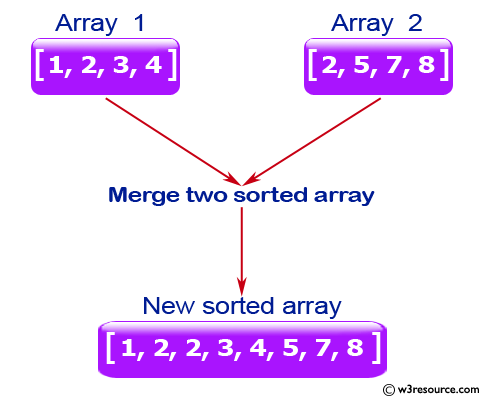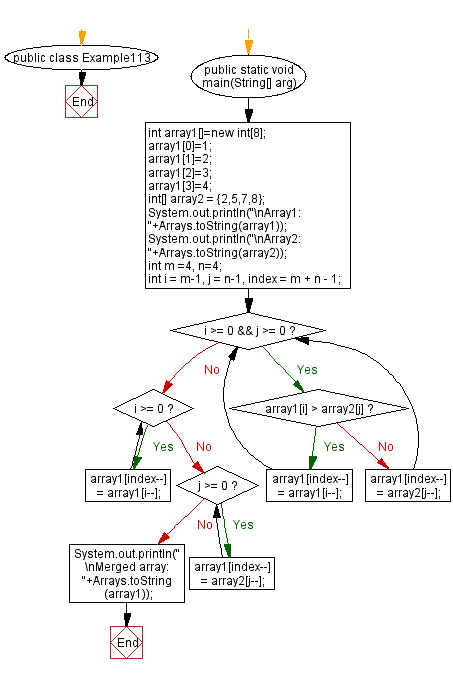﻿ Java: Merge two sorted array and create a new sorted array# Java Exercises: Merge two given sorted array of integers and create a new sorted array

## Java Basic: Exercise-113 with Solution

Write a Java program to merge two given sorted array of integers and create a new sorted array.

Example
array1 = [1,2,3,4]
array2 = [2,5,7, 8]
result = [1,2,2,3,4,5,7,8]

Pictorial Presentation:Sample Solution:

Java Code:

``````import java.util.*;
public class Example113 {
public static void main(String[] arg)
{

// Sorted integer array array1 which has m elements,
// but size of array1 is m+n, sorted integer array array2 which has n elements

//declaration and instantiation.
int array1[]=new int;
//initialization.
array1=1;
array1=2;
array1=3;
array1=4;
int[] array2 = {2,5,7,8};
System.out.println("\nArray1: "+Arrays.toString(array1));
System.out.println("\nArray2: "+Arrays.toString(array2));
int m =4, n=4;
int i = m-1, j = n-1, index = m + n - 1;
while (i >= 0 && j >= 0) {
if (array1[i] > array2[j]) {
array1[index--] = array1[i--];
} else {
array1[index--] = array2[j--];
}
}
while (i >= 0) {
array1[index--] = array1[i--];
}
while (j >= 0) {
array1[index--] = array2[j--];
}

System.out.println("\nMerged array: "+Arrays.toString(array1));
}
}
```
```

Sample Output:

```Array1: [1, 2, 3, 4, 0, 0, 0, 0]

Array2: [2, 5, 7, 8]

Merged array: [1, 2, 2, 3, 4, 5, 7, 8]
```

Flowchart:Java Code Editor:

What is the difficulty level of this exercise?

Test your Programming skills with w3resource's quiz.

﻿

## Java: Tips of the Day

getEnumMap

Converts to enum to Map where key is the name and value is Enum itself.

```public static <E extends Enum<E>> Map<String, E> getEnumMap(final Class<E> enumClass) {
return Arrays.stream(enumClass.getEnumConstants())
.collect(Collectors.toMap(Enum::name, Function.identity()));
}
```

Ref: https://bit.ly/3xXcFZt